### Home > A2C > Chapter Ch4 > Lesson 4.2.2 > Problem4-93

4-93.
1. For each of the following equations, describe how d transforms the parent graph. Homework Help ✎

1. y = dx3

2. y = 3x2d

3. y = (xd)2 + 7

4.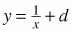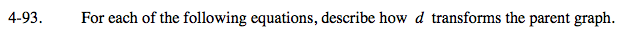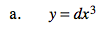Notice that d is being multiplied.

It compresses or streches the graph.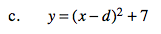What kind of graph does this equation represent?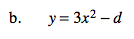Notice that d is being subtracted.

It shifts the graph up or down.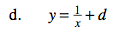See part (b).